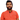# Learn Pain LessHomeOur TeamContact
Flutter
How to Calculate Distance Between Two Locations in FlutterPawneshwer Gupta
March 08, 2023
2 minAt some point, you might need to calculate the distance between two locations in your Flutter application. Whether it’s for tracking the distance traveled by a user, showing the distance between two points on a map, or any other use case, it’s essential to have a reliable and accurate solution.

In this article, we’ll show you how to calculate the distance between two locations in Flutter using the haversine formula, a popular algorithm for calculating distances between two points on a sphere.

Before we dive into the code, let’s first understand what the haversine formula is and how it works.

### .css-c6w1gk{box-sizing:border-box;margin:0;min-width:0;display:block;color:var(--theme-ui-colors-heading,#2d3748);font-weight:bold;-webkit-text-decoration:none;text-decoration:none;margin-bottom:1rem;font-size:1.25rem;position:relative;}What is the Haversine Formula?

The haversine formula is an equation that determines the shortest distance between two points on a sphere, such as the Earth. The formula takes into account the latitude and longitude of the two points and uses trigonometric functions to calculate the distance.

### How does the Haversine Formula Work?

The haversine formula works by first converting the latitude and longitude of the two points from degrees to radians. It then calculates the difference between the latitudes and longitudes and uses the haversine function, which is defined as:

`haversin(x) = sin²(x / 2)`

The haversine function is then used in the main formula, which is:

`distance = 2 * r * arcsin(sqrt(haversin(lat2 - lat1) + cos(lat1) * cos(lat2) * haversin(lon2 - lon1)))`

where r is the radius of the sphere (in kilometers or miles), lat1 and lon1 are the latitude and longitude of the first point, and lat2 and lon2 are the latitude and longitude of the second point.

### Implementing the Haversine Formula in Flutter

Now that we know what the haversine formula is and how it works let’s implement it in Flutter to calculate the distance between two locations.

The first step is to define a function that takes the latitude and longitude of the two points and returns the distance between them. Here’s an example implementation:

```.css-1baulvz{display:inline-block;}import 'dart:math' show cos, sqrt, asin;
double distance(double lat1, double lon1, double lat2, double lon2) {  const r = 6372.8; // Earth radius in kilometers
final a = _haversin(dLat) + cos(lat1Radians) * cos(lat2Radians) * _haversin(dLon);  final c = 2 * asin(sqrt(a));
return r * c;}
double _toRadians(double degrees) => degrees * pi / 180;

The `distance` the function takes four arguments: `lat1`, `lon1`, `lat2`, and `lon2`, which are the latitude and longitude of the two points. The function first converts the latitude and longitude to radians and then calculates the difference between them. It then uses the haversine formula to calculate the distance between the two points.

### Conclusion

Calculating the distance between two locations in Flutter is straightforward using the haversine formula. By implementing the formula in our Flutter application, we can accurately calculate distance between two locations on a sphere.

We hope this article has been helpful in showing you how to calculate the distance between two locations in Flutter. If you have any questions or doubts then comment below.

Start Learning

## 15+TUTORS

We'll send you the best of our blog just once a month. We promise.

Guide

## Software Developer

Pawneshwer Gupta works as a software engineer who is enthusiastic in creating efficient and innovative software solutions.

Python
Flutter
Laravel
NodeJS

## Related PostsHow to Refresh Specific Widget with setState using StatefulBuilder() in Flutter
April 20, 2023
1 min